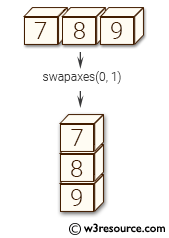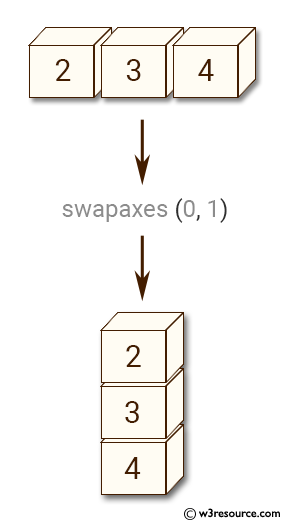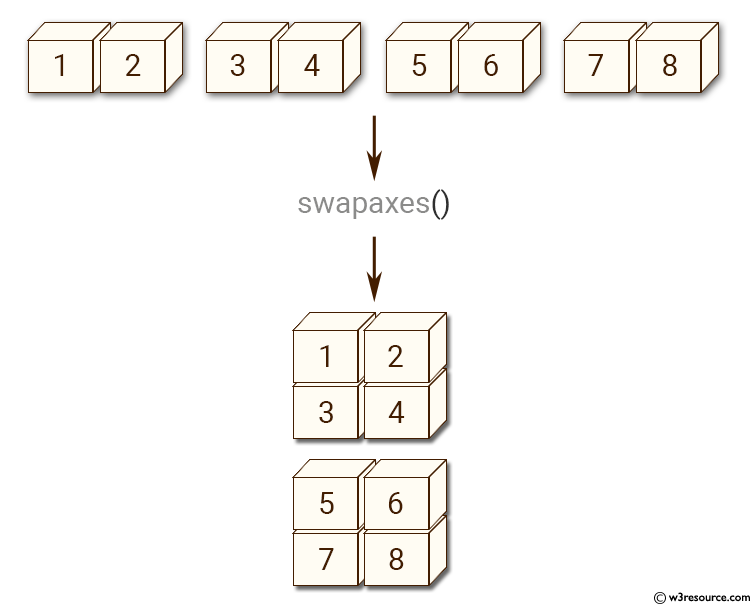# NumPy Array manipulation: swapaxes() function

## numpy.swapaxes() function

The swapaxes() function is used to interchange two axes of an array.

Syntax:

`numpy.swapaxes(a, source, destination)`Version: 1.15.0

Parameter:

Name Description Required /
Optional
a Input array. Required
axis1 First axis. Required
start Second axis. Required

Return value:

a_swapped [ndarray] For NumPy >= 1.10.0, if a is an ndarray, then a view of a is returned; otherwise a new array is created. For earlier NumPy versions a view of a is returned only if the order of the axes is changed, otherwise the input array is returned

Example-1: numpy.swapaxes() function

``````>>> import numpy as np
>>> a = np.array([[2,3,4]])
>>> np.swapaxes(a,0,1)
array([,
,
])
``````

Pictorial Presentation:Example-2: numpy.swapaxes() function

``````>>> import numpy as np
>>> y = np.array([[[1,2],[3,4]],[[5,6],[7,8]]])
>>> y
array([[[1, 2],
[3, 4]],

[[5, 6],
[7, 8]]])
``````

Pictorial Presentation:Example-3: numpy.swapaxes() function

``````>>> import numpy as np
>>> np.swapaxes(y,1,2)
array([[[1, 3],
[2, 4]],

[[5, 7],
[6, 8]]])
``````

Python - NumPy Code Editor:

Previous: rollaxis()
Next: ndarray.T()

﻿